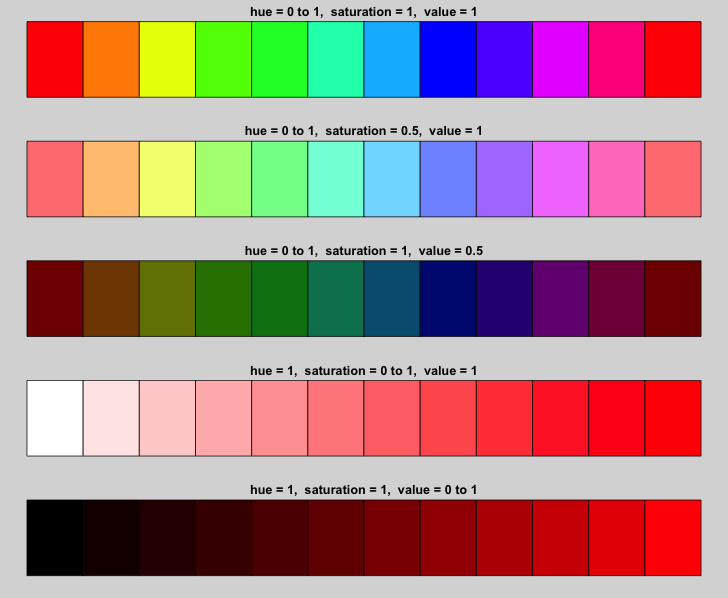# R Graphics: Color Ramp Functions

Color ramp functions in R simplify color selection and assignment.  Visual reference examples are provided to illustrate their use and flexibility.

#### Color Ramp: rainbow()

The rainbow() ramp is is the standard light spectrum with red, green and blue as its base. The functional form is rainbow(n, s=1, v=1, start=0, end=max(1, n-1)/n, alpha=1).  The s and v values correspond to saturation and value from the HCL color scheme (described below).  The parameters `start` and `end` can be used to specify particular subranges of hues. The following values can be used when generating such a subrange: red = 0, yellow = 1/6, green = 2/6, cyan = 3/6, blue = 4/6 and magenta =5/6.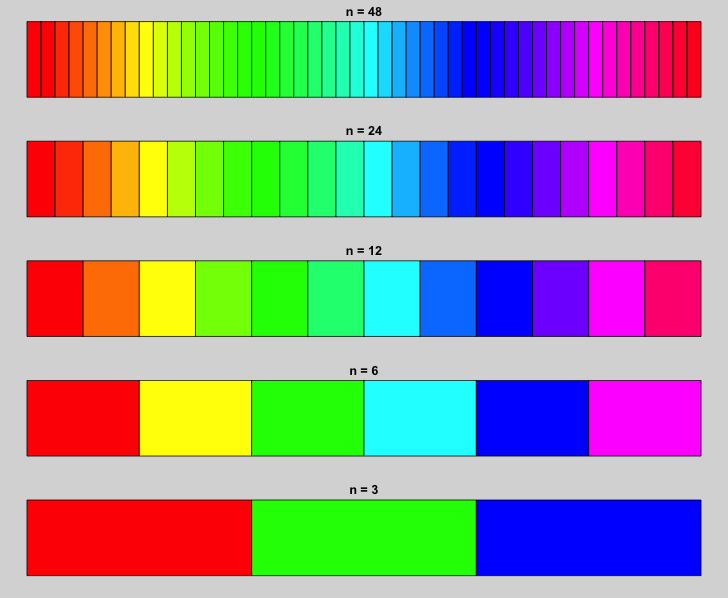#### Color Ramps: terrain.colors()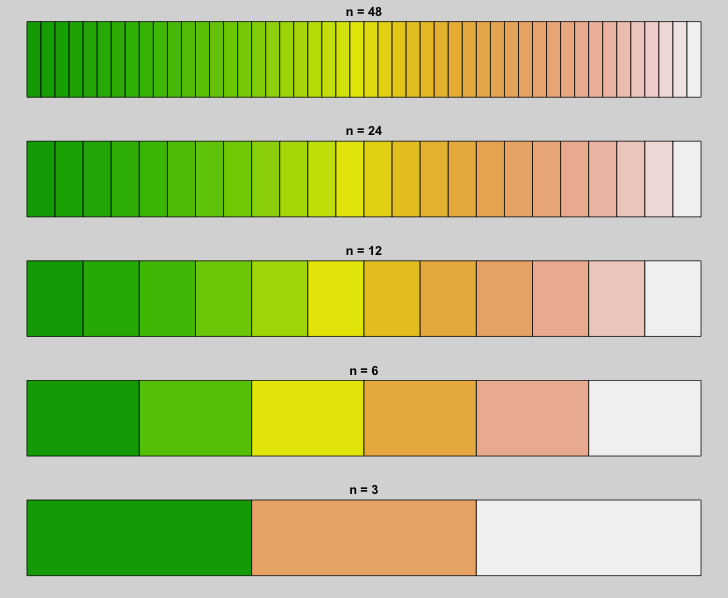#### Color Ramp: topo.colors()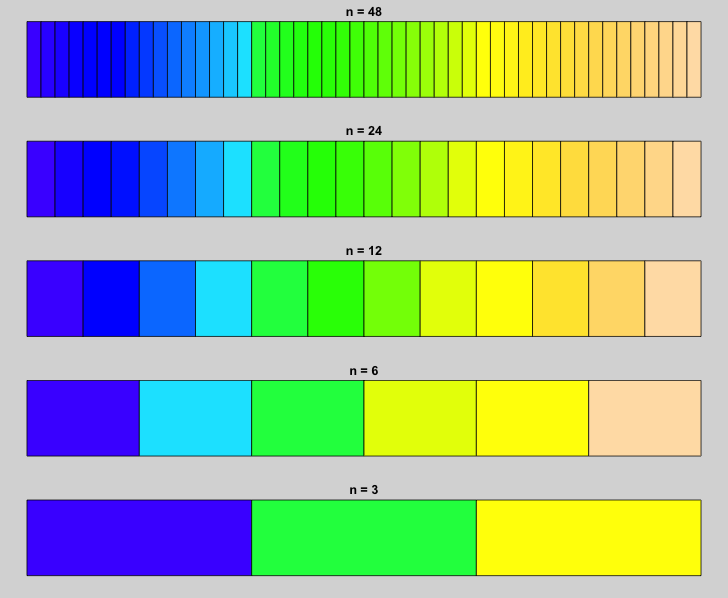#### Color Ramp: cm.colors()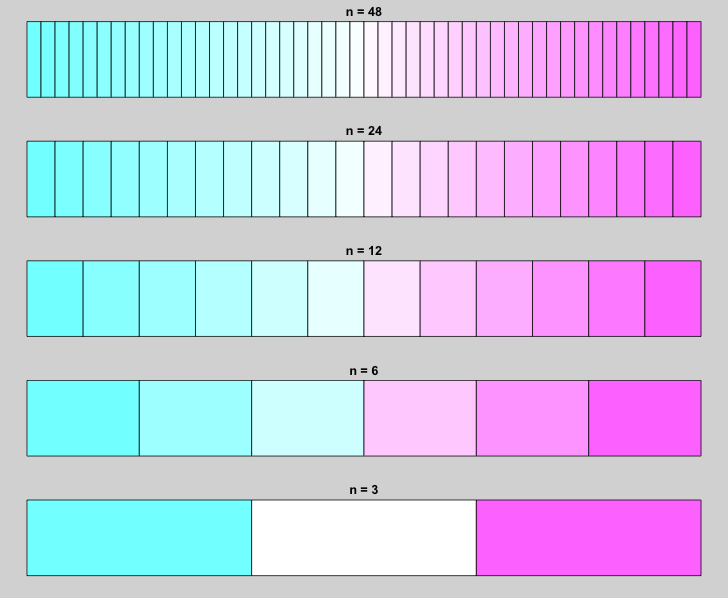#### Color Ramp: hsv()

The hsv() function uses values of hue, saturation, and value (ranging from 0 to 1) to specify a color. The function accepts either a single values or vectors of values, and returns a vector of hexadecimal values.  The function has the form hsv(h=value, s=value, v=value, gamma=value, alpha=value).  In general, hsv() is very flexible and can be used to replicate results from other color ramp functions.